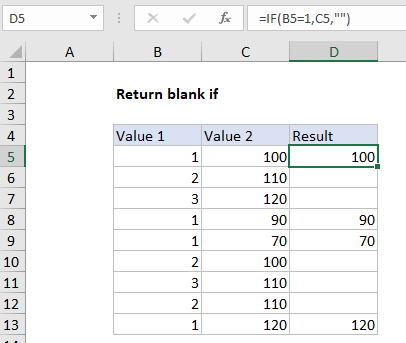## Excel Office

Excel How Tos, Tutorials, Tips & Tricks, Shortcuts

# Return blank if in Excel

This tutorial shows how to calculate Return blank if in Excel using the example below;

# Formula

`=IF(A1=1,B1,"")`## Explanation

To return a blank result using the IF function, you can use an empty string (“”).  In the example shown, the formula in D5 (copied down) is:

`=IF(B5=1,C5,"")`

### How this formula works

This formula is based on the IF function, configured with a simple logical test, a value to return when the test is TRUE, and a value to return when the test is FALSE. In plain English: if Value 1 equals 1, return Value 2. If Value 1 is not 1, return an empty string (“”).

Also See:   How to display conditional message with REPT function in Excel

Note if you type “” directly into a cell in Excel, you’ll see the double quote characters. However, when you enter as a formula like this:

`=""`

You won’t see anything, the cell will look blank.

Also, if you are new to Excel, note numeric values are not entered in quotes. In other words:

```=IF(A1=1,B1,"") // right
=IF(A1="1",B1,"") // wrong```

Wrapping a number in quotes (“1”) causes Excel to interpret the value as text, which will cause logical tests to fail.

Also See:   If this AND that OR that in Excel

### Checking for blank cells

If you need check the result of a formula like this, be aware that the ISBLANK function will return FALSE when checking a formula that returns “” as a final result. There are other options however. If A1 contains “” returned by a formula, then:

```=ISBLANK(A1) // returns FALSE
=COUNTBLANK(A1) // returns 1
=COUNTBLANK(A1)> // returns TRUE```
Also See:   Highlight numbers that include symbols in Excel